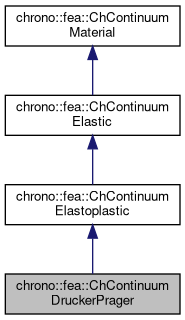chrono::fea::ChContinuumDruckerPrager Class Reference

## Description

Class for the basic properties of elastoplastic materials of Drucker-Prager type, that are useful for simulating soils.

#include <ChContinuumMaterial.h>

Inheritance diagram for chrono::fea::ChContinuumDruckerPrager:[legend]
Collaboration diagram for chrono::fea::ChContinuumDruckerPrager:[legend]

## Public Member Functions

ChContinuumDruckerPrager (double myoung=10000000, double mpoisson=0.4, double mdensity=1000, double melastic_yeld=0.1, double malpha=0.5, double mdilatancy=0)
Create a continuum isotropic Drucker-Prager material.

ChContinuumDruckerPrager (const ChContinuumDruckerPrager &other)

void Set_elastic_yeld (double melastic_yeld)
Set the D-P yield modulus C, for Drucker-Prager yield. More...

double Get_elastic_yeld () const
Get the elastic yield modulus C.

void Set_alpha (double malpha)
Set the internal friction coefficient A.

double Get_alpha () const
Get the internal friction coefficient A.

void Set_from_MohrCoulomb (double phi, double cohesion, bool inner_approx=true)
Sets the C and A parameters of the Drucker-Prager model starting from more 'practical' values of inner friction angle phi and cohesion, as used in the faceted Mohr-Coulomb model. More...

virtual void Set_flow_rate (double mflow_rate) override
Set the plastic flow rate multiplier. More...

virtual double Get_flow_rate () const override
Get the flow rate multiplier.

void Set_dilatancy (double mdilatancy)
Set the internal dilatation coefficient (usually 0.. < int.friction)

double Get_dilatancy () const
Get the internal dilatation coefficient.

void Set_hardening_limit (double mhl)
Set the hardening limit (usually a bit larger than yield), or softening.

double Get_hardening_limit () const
Get the hardening limit.

void Set_hardening_speed (double mhl)
Set the hardening inverse speed coeff. More...

double Get_hardening_speed () const
Get the hardening speed.

virtual double ComputeYeldFunction (const ChStressTensor<> &mstress) const override
Return a scalar value that is 0 on the yield surface, <0 inside (elastic), >0 outside (incompatible->plastic flow)

virtual void ComputeReturnMapping (ChStrainTensor<> &mplasticstrainflow, const ChStrainTensor<> &mincrementstrain, const ChStrainTensor<> &mlastelasticstrain, const ChStrainTensor<> &mlastplasticstrain) const override
Correct the strain-stress by enforcing that elastic stress must remain on the yield surface, computing a plastic flow to be added to plastic strain while integrating.

virtual void ComputePlasticStrainFlow (ChStrainTensor<> &mplasticstrainflow, const ChStrainTensor<> &mestrain) const override
Compute plastic strain flow direction from strain according to Drucker-Prager.

virtual void ArchiveOUT (ChArchiveOut &marchive) override

virtual void ArchiveIN (ChArchiveIn &marchive) overridePublic Member Functions inherited from chrono::fea::ChContinuumElastoplastic
ChContinuumElastoplastic (double myoung=10000000, double mpoisson=0.4, double mdensity=1000)Public Member Functions inherited from chrono::fea::ChContinuumElastic
ChContinuumElastic (double myoung=10000000, double mpoisson=0.4, double mdensity=1000)
Create a continuum isotropic hookean material. More...

ChContinuumElastic (const ChContinuumElastic &other)

void Set_E (double m_E)
Set the Young E elastic modulus, in Pa (N/m^2), as the ratio of the uniaxial stress over the uniaxial strain, for hookean materials. More...

double Get_E () const
Get the Young E elastic modulus, in Pa (N/m^2).

void Set_v (double m_v)
Set the Poisson v ratio, as v=-transverse_strain/axial_strain, so takes into account the 'squeezing' effect of materials that are pulled (so, if zero, when you push the two sizes of a cube, it won't inflate). More...

double Get_v () const
Get the Young v ratio, as v=-transverse_strain/axial_strain.

void Set_G (double m_G)
Set the shear modulus G, in Pa (N/m^2), as the ratio of shear stress to the shear strain. More...

double Get_G () const
Get the shear modulus G, in Pa (N/m^2)

double Get_l () const
Get Lamé first parameter (the second is shear modulus, so Get_G() )

double Get_BulkModulus () const
Get bulk modulus (increase of pressure for decrease of volume), in Pa.

double Get_WaveModulus () const
Get P-wave modulus (if V=speed of propagation of a P-wave, then (M/density)=V^2 )

void ComputeStressStrainMatrix ()
Computes Elasticity matrix and stores the value in this->StressStrainMatrix Note: is performed every time you change a material parameter.

ChMatrixDynamicGet_StressStrainMatrix ()
Get the Elasticity matrix.

void ComputeElasticStress (ChStressTensor<> &mstress, const ChStrainTensor<> &mstrain) const
Compute elastic stress from elastic strain (using column tensors, in Voight notation)

void ComputeElasticStrain (ChStrainTensor<> &mstrain, const ChStressTensor<> &mstress) const
Compute elastic strain from elastic stress (using column tensors, in Voight notation)

void Set_RayleighDampingM (double m_d)
Set the Rayleigh mass-proportional damping factor alpha, to build damping R as R=alpha*M + beta*K.

double Get_RayleighDampingM () const
Set the Rayleigh mass-proportional damping factor alpha, in R=alpha*M + beta*K.

void Set_RayleighDampingK (double m_d)
Set the Rayleigh stiffness-proportional damping factor beta, to build damping R as R=alpha*M + beta*K.

double Get_RayleighDampingK () const
Set the Rayleigh stiffness-proportional damping factor beta, in R=alpha*M + beta*K.Public Member Functions inherited from chrono::fea::ChContinuumMaterial
ChContinuumMaterial (double mdensity=1000)

ChContinuumMaterial (const ChContinuumMaterial &other)

void Set_density (double m_density)
Set the density of the material, in kg/m^2.

double Get_density () const
Get the density of the material, in kg/m^2.Protected Attributes inherited from chrono::fea::ChContinuumMaterial
double density

## ◆ Set_elastic_yeld()

 void chrono::fea::ChContinuumDruckerPrager::Set_elastic_yeld ( double melastic_yeld )
inline

Set the D-P yield modulus C, for Drucker-Prager yield.

It defines the transition elastic->plastic.

## ◆ Set_flow_rate()

 virtual void chrono::fea::ChContinuumDruckerPrager::Set_flow_rate ( double mflow_rate )
inlineoverridevirtual

Set the plastic flow rate multiplier.

The lower the value, the slower the plastic flow during dynamic simulations.

Implements chrono::fea::ChContinuumElastoplastic.

## ◆ Set_from_MohrCoulomb()

 void chrono::fea::ChContinuumDruckerPrager::Set_from_MohrCoulomb ( double phi, double cohesion, bool inner_approx = true )

Sets the C and A parameters of the Drucker-Prager model starting from more 'practical' values of inner friction angle phi and cohesion, as used in the faceted Mohr-Coulomb model.

Use the optional parameter inner_approx to set if the faceted Mohr-Coulomb must be approximated with D-P inscribed (default) or circumscribed.

## ◆ Set_hardening_speed()

 void chrono::fea::ChContinuumDruckerPrager::Set_hardening_speed ( double mhl )
inline

Set the hardening inverse speed coeff.

for exponential hardening (the larger, the slower the hardening or softening process that will asymptotically make yield = hardening_limit )

The documentation for this class was generated from the following files:
• /builds/uwsbel/chrono/src/chrono/fea/ChContinuumMaterial.h
• /builds/uwsbel/chrono/src/chrono/fea/ChContinuumMaterial.cpp